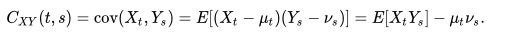# Cross Covariance

Cross covariance of x and y, in statistics, is a measure of the similarity between x and shifted versions of y, as a function of the shift (lag). The cross covariance is given by the equationwhere E is the expectation operator, and the processes have mean functions vt=E[Yt] and μt=E[Xt]

In signal processing, cross-covariance has a slightly different definition: it measures the similarity between two signals, and is a function of the time between signals. It is sometimes called the sliding dot product or the cross-correlation.

Note that, in statistics, the cross-correlation and cross covariance are related but are not the same thing.

CITE THIS AS:
Stephanie Glen. "Cross Covariance" From StatisticsHowTo.com: Elementary Statistics for the rest of us! https://www.statisticshowto.com/cross-covariance/
---------------------------------------------------------------------------Need help with a homework or test question? With Chegg Study, you can get step-by-step solutions to your questions from an expert in the field. Your first 30 minutes with a Chegg tutor is free!